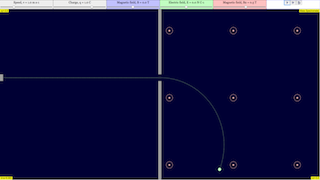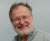Introduction

## Mass spectrometry

 This is the simulation of the motion of a mass m situated at the end of a spring of length l and negligible mass. The motion is restricted to the horizontal dimension. (We choose a coordinate system in the plane with origin at the fixed end of the spring and with the X axis along the direction of the spring).

We assume that the reaction of the spring to a displacement dx from the equilibrium point can be modeled using Hooke's Law, F(dx) = -k dx , where k is a constant which depends on the physical characteristics of the spring. Thus, applying Newton's Second Law, we obtain the following second-order ordinary differential equation:

d2x / dt2 = -k/m (x-l),

where x is the horizontal position of the free end of the spring.

In the simulation we solve numerically this equation and visualize the results.

Activities

## Activities

1. Measure the period of the motion for the given initial conditions.
2. Drag with the mouse the ball to a new position and measure the period again. What do you observe?
3. Set the mass of the ball to several different values (keeping k constant) and plot in your notebook the observed period versus the mass.
4. Do the same for the elastic constant of the spring, k.
5. Would you dare to provide an explicit formula for the dependence of the period with respect to the mass and k?
6. Should the total energy of the model be preserved?
7. Why do you think the total energy of the simulation slowly increases? (Hint: choose a better solver for the equations, such as Runge-Kutta, and check again.)

### Translations

Code Language Translator Run### Software Requirements

SoftwareRequirements

 Android iOS Windows MacOS with best with Chrome Chrome Chrome Chrome support full-screen? Yes. Chrome/Opera No. Firefox/ Samsung Internet Not yet Yes Yes cannot work on some mobile browser that don't understand JavaScript such as..... cannot work on Internet Explorer 9 and below

### CreditsWolfgang Christian; Francisco Esquembre

[text]